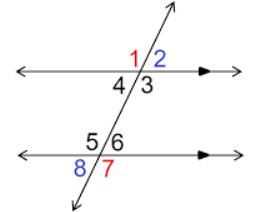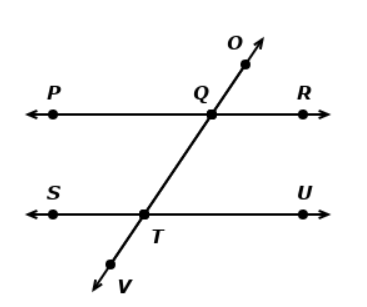# Different Types Of Angles And their Properties

This quiz contains 10 multiple-choice questions. The quiz covers the topics related to the different types of angles as well as their properties.

Start Quiz

Which of the given angles are interior alternate angles?(Select all that apply)Angle 5 and Angle 2

Angle 3 and Angle 5

Angle 4 and Angle 6

Angle 3 and Angle 6

Which of the given angles are exterior alternate angles? (Select all that apply)Angle 8 and Angle 2

Angle 4 and Angle 7

Angle 4 and Angle 2

Angle 1 and Angle 5

Which of the given angles are vertically opposite angles? (Select all that apply)Angle 6 and Angle 5

Angle 3 and Angle 6

Angle 2 and Angle 4

Angle 6 and Angle 2

The lines PR is parallel to SU are parallel to each other.

Choose the correct pair of interior alternate angles.Angle PQO and Angle OQR

Angle QTU and Angle QTS

Angle STQ and Angle PQT

Angle RQT and Angle QTS

The lines PR is parallel to SU are parallel to each other.

Choose the correct pair that forms supplementary angles.(Select all that apply)Angle PQO and Angle OQR

Angle QTU and Angle QTS

Angle STQ and Angle PQO

Angle RQT and Angle STQ

Find angle 1 if angle 5 is 120 degrees.120 degrees

60 degrees

150 degrees

If angle 6 is 50 degrees, find the value of angle 1.50 degrees

130 degrees

80 degrees

The lines PR is parallel to SU are parallel to each other.

Find angle PQO if the angle QTU is 60 degrees.Angle PQO = ##60## degrees

60

The lines PR is parallel to SU are parallel to each other.

Find the value of angle STV if the angle RQT is 135 degrees.Angle STV = ##45## degrees

45

The lines PR is parallel to SU are parallel to each other.

Choose the correct pair of corresponding angles. (Select all that apply)Angle PQO and Angle STQ

Angle QTU and Angle OQR

Angle STQ and Angle PQT

Angle RQT and Angle UTQ

Quiz/Test Summary
Title: Different Types Of Angles And their Properties
Questions: 10
Contributed by: